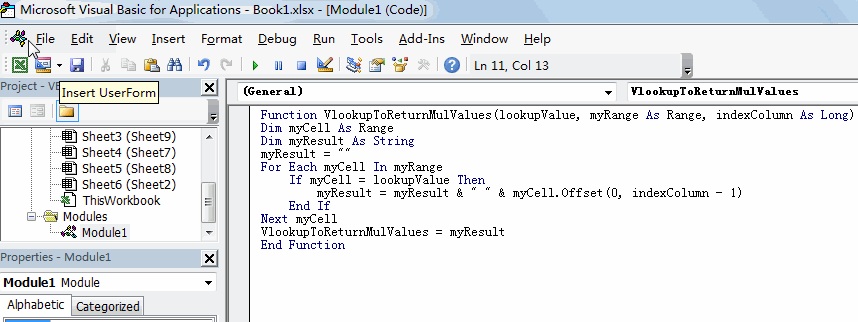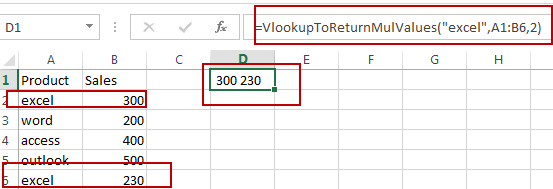# How to Vlookup to Return Multiple Values in Excel

This post will guide you how to vlookup to return multiple values in one cell in Excel. How do I lookup and return multiple values concatenated into one cell with an User defined function in Excel.

## Vlookup to Return Multiple Values in One Cell

Assuming that you have a list of data in range A1:B6, and you would like to use a vlookup that looks up the value “excel” in column A and returns a concatenated string of all the matched values in column B.

For example, If I was looking up “excel” value, it should return “300 230” as one text string. How to do it. The default VLOOKUP function or INDEX/MATCH functions cannot be used to resolve this problem. And you have to create an User Defined Function with VBA code to achieve the result. Here are the steps:

#1 open your excel workbook and then click on “Visual Basic” command under DEVELOPER Tab, or just press “ALT+F11” shortcut.#2 then the “Visual Basic Editor” window will appear.

#3 click “Insert” ->”Module” to create a new module.#4 paste the below VBA code into the code window. Then clicking “Save” button.#5 back to the current worksheet, then type the following formula in a blank cell, and then press Enter key.

=VlookupToReturnMulValues(“excel”,A1:B6,2)

#6 you should notice that multiple values has been returned and concatenated as one text string in one cell.Related Posts

How to Extract First Letter from Each Word in a Cell in Excel

This post will guide you how to extract first letter from each word in a given cell in Excel. How do I extract the first letter of each word in a range of names in your current worksheet in Microsoft ...

How to ignore Blank Cells while Concatenating cells in Excel

This post will guide you how to concatenate cells but ignore all blank cells in your worksheet in Excel. How do I concatenate cells but ignore blank cells with a formula in Excel. How to create a concatenate formula to ...

How to Calculate the Average Excluding the Smallest & Highest Numbers in Excel

Calculating the average for a batch of data is frequently used in our daily life. But for some cases like statistic the average score in a competition, or price analysis, we often calculate the average excluding the smallest and highest ...

How to Get the Maximum or Minimum Absolute Value in Excel

It is easy to find the maximum or minimum value in a batch of data in excel, but if this batch of numbers contains both positive and negative numbers, the maximum or minimum absolute value cannot be found out by ...

How to Count Only Numbers in Bold in a Range of Cells in Excel

This post will guide you how to Count only numbers with bold style in a range of cells in Excel. How do I Count on cells with bold font within a range of cells using User defined function in Excel ...

Sidebar